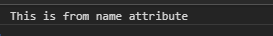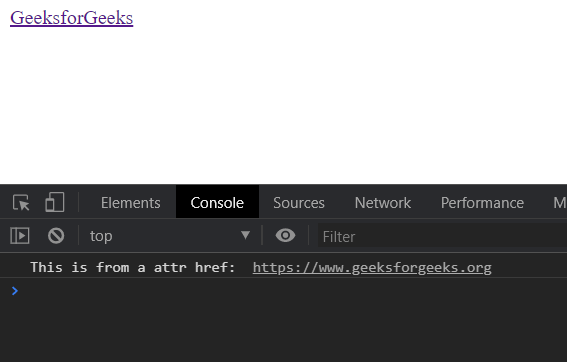Related Articles

# D3.js selection.attr() Function

• Last Updated : 19 Aug, 2020

The selection.attr() function is used to set the attribute of the element that is selected. The name of the attribute and value of the attributes are to be set using this function.

Syntax:

Hey geek! The constant emerging technologies in the world of web development always keeps the excitement for this subject through the roof. But before you tackle the big projects, we suggest you start by learning the basics. Kickstart your web development journey by learning JS concepts with our JavaScript Course. Now at it's lowest price ever!

```selection.attr(name[, value]);
```

Parameters: This function takes two parameters as shown above and described below:

• name: It is the name of the attribute.
• value: It is the value of the attribute.

Return Values: This function does not return any value.

Example 1:

## HTML

 ```<``html` `lang``=``"en"``>`` ` `<``head``>``    ``<``meta` `charset``=``"UTF-8"``>``    ``<``meta` `name``=``"viewport"` `path1tent``=``        ``"width=device-width,initial-scale=1.0"``>``         ` `    ``<``title``>D3.js selection.attr() Function`` ` `    ``<``script` `src``=``        ``"https://d3js.org/d3.v4.min.js"``>``    ```` ` `    ``<``style``>``        ``p {``            ``line-height: 5px;``        ``}``    `````` ` `<``body``>``    ``<``div``>``        ``<``p``>GeeksforGeeks``    ```` ` `    ``<``script``>``        ``var div = d3.select("p")``            ``.attr("name", "This is from name attribute");``        ``var divselect = document.querySelector("p");``        ``console.log(divselect.getAttribute("name"));``    `````` ` ``

Output:Example 2:

## HTML

 ```<``html` `lang``=``"en"``>`` ` `<``head``>``    ``<``meta` `charset``=``"UTF-8"``>``    ``<``meta` `name``=``"viewport"` `path1tent``=``        ``"width=device-width,initial-scale=1.0"``>`` ` `    ``<``title``>D3.js selection.attr() Function`` ` `    ``<``script` `src``=``        ``"https://d3js.org/d3.v4.min.js"``>``    ```` ` `    ``<``style``>``        ``p {``            ``line-height: 5px;``        ``}``    `````` ` `<``body``>``    ``<``div``>``        ``<``a``>GeeksforGeeks``    ````     ` `    ``<``script``>``        ``var a = d3.select("a").attr("href", ``            ``"https://www.geeksforgeeks.org");`` ` `        ``var divselect = document.querySelector("a");``         ` `        ``console.log("This is from a attr href: ", ``                ``divselect.getAttribute("href"));``    `````` ` ``

Output:My Personal Notes arrow_drop_up